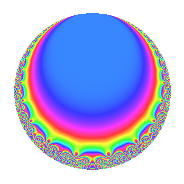# Properties

 Label 20.4.cLevel 20 Weight 4 Character orbit c Rep. character $$\chi_{20}(9,\cdot)$$ Character field $$\Q$$ Dimension 2 Newforms 1 Sturm bound 12 Trace bound 0

# Related objects

## Defining parameters

 Level: $$N$$ = $$20 = 2^{2} \cdot 5$$ Weight: $$k$$ = $$4$$ Character orbit: $$[\chi]$$ = 20.c (of order $$2$$ and degree $$1$$) Character conductor: $$\operatorname{cond}(\chi)$$ = $$5$$ Character field: $$\Q$$ Newforms: $$1$$ Sturm bound: $$12$$ Trace bound: $$0$$

## Dimensions

The following table gives the dimensions of various subspaces of $$M_{4}(20, [\chi])$$.

Total New Old
Modular forms 12 2 10
Cusp forms 6 2 4
Eisenstein series 6 0 6

## Trace form

 $$2q$$ $$\mathstrut +\mathstrut 14q^{5}$$ $$\mathstrut -\mathstrut 98q^{9}$$ $$\mathstrut +\mathstrut O(q^{10})$$ $$2q$$ $$\mathstrut +\mathstrut 14q^{5}$$ $$\mathstrut -\mathstrut 98q^{9}$$ $$\mathstrut +\mathstrut 40q^{11}$$ $$\mathstrut +\mathstrut 152q^{15}$$ $$\mathstrut -\mathstrut 168q^{19}$$ $$\mathstrut +\mathstrut 152q^{21}$$ $$\mathstrut -\mathstrut 54q^{25}$$ $$\mathstrut +\mathstrut 12q^{29}$$ $$\mathstrut -\mathstrut 448q^{31}$$ $$\mathstrut -\mathstrut 152q^{35}$$ $$\mathstrut +\mathstrut 912q^{39}$$ $$\mathstrut +\mathstrut 532q^{41}$$ $$\mathstrut -\mathstrut 686q^{45}$$ $$\mathstrut +\mathstrut 534q^{49}$$ $$\mathstrut -\mathstrut 1216q^{51}$$ $$\mathstrut +\mathstrut 280q^{55}$$ $$\mathstrut -\mathstrut 56q^{59}$$ $$\mathstrut +\mathstrut 364q^{61}$$ $$\mathstrut -\mathstrut 912q^{65}$$ $$\mathstrut -\mathstrut 1064q^{69}$$ $$\mathstrut +\mathstrut 816q^{71}$$ $$\mathstrut +\mathstrut 2128q^{75}$$ $$\mathstrut +\mathstrut 96q^{79}$$ $$\mathstrut +\mathstrut 698q^{81}$$ $$\mathstrut +\mathstrut 1216q^{85}$$ $$\mathstrut -\mathstrut 3052q^{89}$$ $$\mathstrut -\mathstrut 912q^{91}$$ $$\mathstrut -\mathstrut 1176q^{95}$$ $$\mathstrut -\mathstrut 1960q^{99}$$ $$\mathstrut +\mathstrut O(q^{100})$$

## Decomposition of $$S_{4}^{\mathrm{new}}(20, [\chi])$$ into irreducible Hecke orbits

Label Dim. $$A$$ Field CM Traces $q$-expansion
$$a_2$$ $$a_3$$ $$a_5$$ $$a_7$$
20.4.c.a $$2$$ $$1.180$$ $$\Q(\sqrt{-19})$$ None $$0$$ $$0$$ $$14$$ $$0$$ $$q-\beta q^{3}+(7+\beta )q^{5}+\beta q^{7}-7^{2}q^{9}+\cdots$$

## Decomposition of $$S_{4}^{\mathrm{old}}(20, [\chi])$$ into lower level spaces

$$S_{4}^{\mathrm{old}}(20, [\chi]) \cong$$ $$S_{4}^{\mathrm{new}}(10, [\chi])$$$$^{\oplus 2}$$